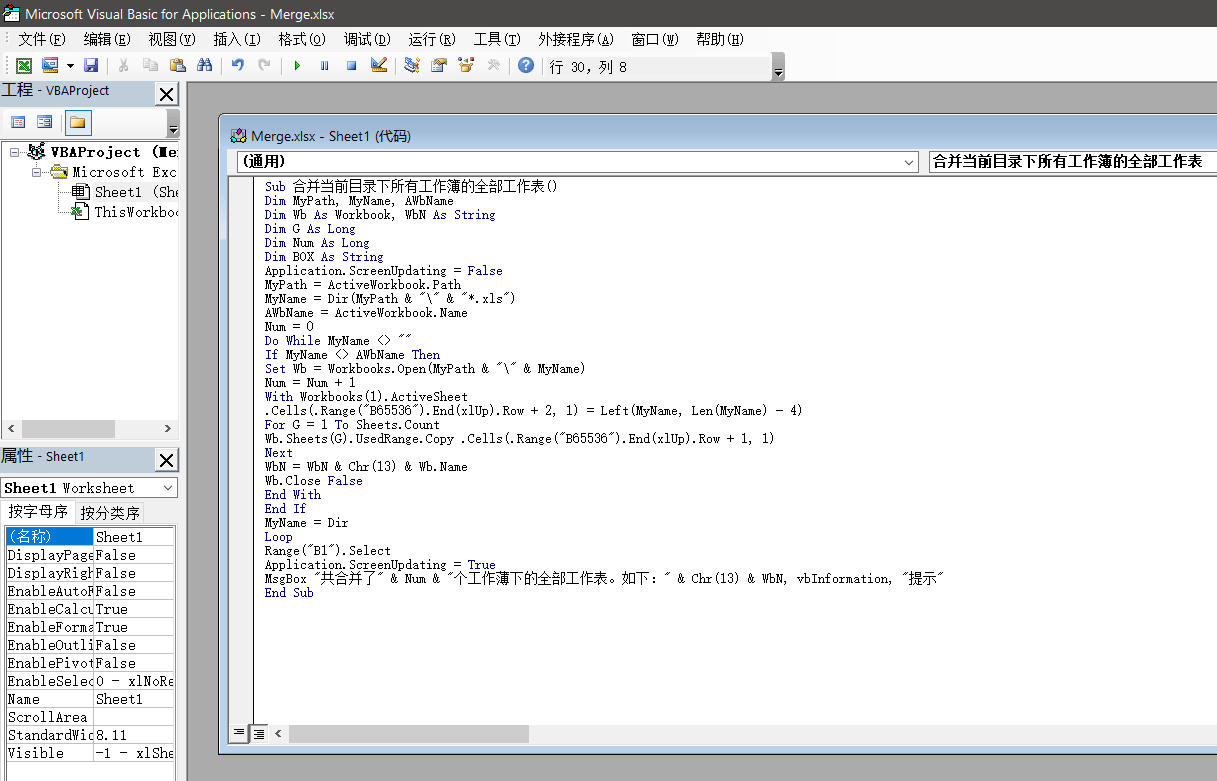# 如何合并多个 Excel 表格文件至一个总表格

1. 把需要合并的多个 Excel 表格放在同一文件夹内，并在该文件夹下新建一个 Excel 文件。（图中以 Merge.xlsx 举例）2. Microsoft Excel 打开刚刚新建的 Excel 文件 Merge.xlsx ，在表格下方右键单击 sheet1 ，选择 查看代码 。点击后进入宏计算界面。3. 在代码窗口中输入以下代码：

Sub 合并当前目录下所有工作簿的全部工作表()
Dim MyPath, MyName, AWbName
Dim Wb As Workbook, WbN As String
Dim G As Long
Dim Num As Long
Dim BOX As String
Application.ScreenUpdating = False
MyPath = ActiveWorkbook.Path
MyName = Dir(MyPath & "\" & "*.xls")
AWbName = ActiveWorkbook.Name
Num = 0
Do While MyName <> ""
If MyName <> AWbName Then
Set Wb = Workbooks.Open(MyPath & "\" & MyName)
Num = Num + 1
With Workbooks(1).ActiveSheet
.Cells(.Range("B65536").End(xlUp).Row + 2, 1) = Left(MyName, Len(MyName) - 4)
For G = 1 To Sheets.Count
Wb.Sheets(G).UsedRange.Copy .Cells(.Range("B65536").End(xlUp).Row + 1, 1)
Next
WbN = WbN & Chr(13) & Wb.Name
Wb.Close False
End With
End If
MyName = Dir
Loop
Range("B1").Select
Application.ScreenUpdating = True
MsgBox "共合并了" & Num & "个工作薄下的全部工作表。如下：" & Chr(13) & WbN, vbInformation, "提示"
End Sub4. 在上方菜单栏点击 运行 - 运行子过程/用户窗体5. 点击 运行 之后稍作等待，等待时间大部分取决于表格数量。运行完毕后会提示已合并 x 个文件，点击确定即可查看、保存合并后的表格，该表格包括同文件夹下所有表格文件的内容。